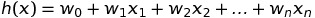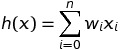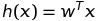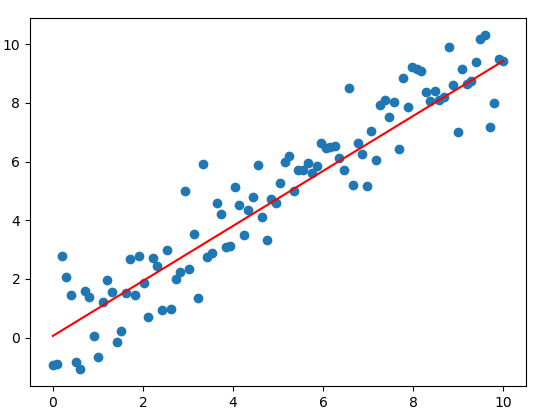# Learn TensorFlow: Linear Regression

· AI Zone · Tutorial
Save
10.40K Views

## Introduction to Linear Regression

An important algorithm of supervised learning is linear regression. In this article, I am going to re-use the following notations that I have referred from  (in the References section).

• xi denotes the “input” variables, also called input features
• yi denotes the “ouput” or target variable that we are trying to predict
• A pair (xi, yi) is called a training example
• A list of m training examples {xi, yi; i = 1,…,m} is called a training set
• The superscript “i” in the notation is an index into the training set
• X denotes the space of input values and Y denotes the space of output values. In this article, I am going to assume that X = Y = R
• A function h: X -> Y, where h(x) is a good predictor for the corresponding value of y, is called a hypothesis or a model

When the target variable that we are trying to predict is continuous, we call the learning problem a regression problem. When y takes on only a small number of discrete values, we call it a classification problem.

Linear regression means you can add up the inputs multiplied by some constants to get output and we are going to represent h function as follows:Where the wi’s are the parameters (also called weights) parameterizing the space of linear functions mapping from X to Y. To simplicity, we also assume that x­0 = 1 and our h(x) can look like this:If we view w and x both as vectors, we can re-write h(x):Where x = (x0, x1, x2,…,xn) and w = (w0, w1,…,wn).

So far, a question is going to occur, which is, how can we get the weights w? To answer this question, we are going to define a cost function that is used to compute error as the difference between predicted h(x) and the actual y. The cost function looks like this:We want to choose w so as to minimize costF(w). To do this, we are going to use a gradient descent algorithm. In this way, we repeatedly run through the training set, and each time we encounter a training example, we update the weights according to the gradient of the error with respect to that single training example only.

## Using TensorFlow

#### Initializing a Linear Model

In this article, I assume that our model (or h function) is the following equation:

`h(x) = w1*x + w0, where x0 = 1, x1 = x`

Initializing a Training Set

We need to initialize data by creating the following Python script:

``````import numpy as np

import matplotlib.pyplot as plt

x_train = np.linspace(0, 10, 100)

y_train = x_train + np.random.normal(0,1,100)

plt.scatter(x_train, y_train)

plt.show()``````

If you run this script, the result can look like this:After initializing the training set, we repeatedly run through the training set, and each time we encounter a training example, we update the weights according to the gradient of the error with respect to that single training example only. The following code will allow you to create a best-fit line for the given data by using TensorFlow library:

``````import tensorflow as tf

import numpy as np

import matplotlib.pyplot as plt

learning_rate = 0.01

# steps of looping through all your data to update the parameters

training_epochs = 100

# a training set

x_train = np.linspace(0, 10, 100)

y_train = x_train + np.random.normal(0,1,100)

# set up placeholders for input and output

X = tf.placeholder(tf.float32)

Y = tf.placeholder(tf.float32)

# Define h(x) = x*w1 + w0

def h(X, w1, w0):

# set up variables for weights

w0 = tf.Variable(0.0, name="weights")

w1 = tf.Variable(0.0, name="weights")

y_predicted = h(X, w1, w0)

# Define the cost function

costF = 0.5*tf.square(Y-y_predicted)

# Define the operation that will be called on each iteration

sess = tf.Session()

init = tf.global_variables_initializer()

sess.run(init)

# Loop through the data training

for epoch in range(training_epochs):

for (x, y) in zip(x_train, y_train):

sess.run(train_op, feed_dict={X: x, Y: y})

# get values of the final weights

w_val_0 = sess.run(w0)

w_val_1 = sess.run(w1)

sess.close()

# plot the data training

plt.scatter(x_train, y_train)

# plot the best fit line

y_learned = x_train*w_val_1 + w_val_0

plt.plot(x_train, y_learned, 'r')

plt.show()``````

The result of running the script above:## Conclusion

In this article, I introduced how to solve a linear regression problem by using a gradient descent algorithm. One problem with linear regression is that it tends to underfit the data, and one way to solve this problem is a technique known as locally weighted linear regression. You can discover more about this technique in .

## References

 Machine Learning in Action by Peter Harrington

 Machine Learning with TensorFlow by Nishant Shukla

 TensorFlow Machine Learning Cookbook by Nick McClure

 Data Science from Scratch by Joel Grus

 Hands-on Machine Learning with Scikit-Learn & TensorFlow by Aurélien Géron